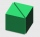# Mathematics

Is there a lousy classification of mathematics or certification or math exam?
Do you want to become more accustomed to it and transform the boring subject into a fun exploration?
Want to feel the joy of understanding and computing an example?
Do you want to train in thinking, looking for problems solutions?
We consider mathematics to be a bearer of common sense, without which t isn't straightforward in the next career to achieve success. Whether as a manager, programmer, judge, mason, or electrician, you will make decisions every day, the quality of which will depend on your wisdom, maturity, concentration. The smartest and better decisions you make in life, the greater the potential for success in your personal and working life. This will help you with our portal with examples of mathematics with their solutions. If you are looking for math material, you are getting ready for a prize, a graduate, a monitor, a nationwide knowledge test is also right here. In addition to examples, we also offer online math calculators.

• Two chordsIn a circle with radius r = 26 cm two parallel chords are drawn. One chord has a length t1 = 48 cm and the second has a length t2 = 20 cm, with the center lying between them. Calculate the distance of two chords.
• 3/8 offA shirt cost 38.95 How much will you pay for the shirt if it has a discount tag for 3/8 off? Round to the nearest cent
• Rectangular base pyramidCalculate an area of the shell of the pyramid with a rectangular base of 2.8 m and 1.4 m and height 2.5 meters.
• Wall heightCalculate the height of a regular hexagonal pyramid with a base edge of 5 cm and a wall height w = 20 cm.
• Hexa pyramidThe base of the regular pyramid is a hexagon, which can be described by a circle with a radius of 1 m. Find the volume of the pyramid 2.5 m high.
• Triangular prismCalculate the surface of a triangular prism with the base of an equilateral triangle with a side length of 7.5 cm and a corresponding height of 6.5 cm. Prism height is 15cm.
• DivideHow many different ways can three people divide 7 pears and 5 apples?